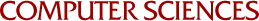On Two Polynomial Spaces Associated With a Box Spline

Amos Ron, Carl deBoor and Nira Dyn
1989

The polynomial space H in the span of the integer translates of a box spline M admits a well-known characterization as the joint kernel of a set of homogeneous differential operators with constant coefficients. The dual space H* has a convenient representation by a polynomial space P, explicitly known, which plays an important role in box spline theory as well as in multivariate polynomial interpolation. In this paper we characterize the dual space P as the joint kernel of simple differential operators, each one a power of a directional derivative. Various applications of this result to multivariate polynomial interpolation, multivariate splines and duality between polynomial and exponential spaces are discussed.